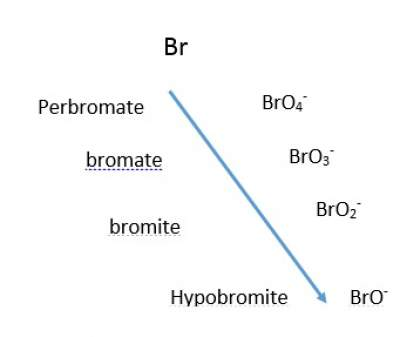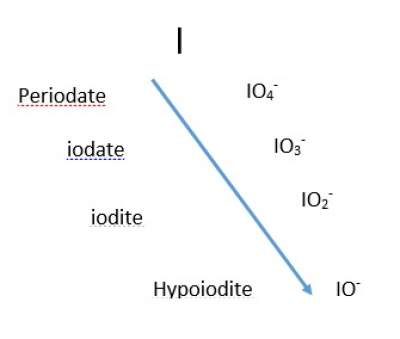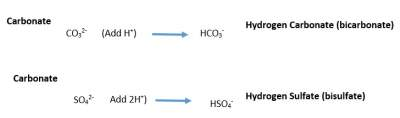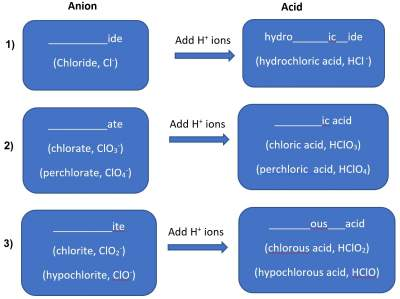# Nomenclature of Compounds

### Cations

• Monoatomic (with one charge such as Na+; two charges such as Mg2+); three charges such as Fe3+;
• Polyatomic composed of two or more atoms, for example, NH3
Except the polyatomic cations, all cations are formed form metal atomswhich are located at the left hand side of the periodic table

### Anions

• Monoatomic (with one charge such as H; two charges such as S2−)
• Polyatomic with oxygen (Oxyanions)
• Single-member anios where -ate is added to the name to the non-oxygen atom, for example Permanganate ion MnO4; Carbonate ion CO 32−
• Two-member anions with more oxygens get the suffix "ate", and the ones with fewer oxygens get the suffix "ite".
For example, Nitrite NO2;Nitrate NO3
• Four-member anions follow pattern as shown below regarding the prefix and suffix,• Polyatomic with oxygen & Hydrogen: Add hydrogen- or digydrogen- to the anion's name depending on the number of H+ added. Examples are shown below:### Ionic Compounds

By simply adding the name of the Cation to the name of the Anion.
Examples:
Calcium Chloride: CaCl2; Alumninum Nitrate Al(NO3)3; Ammonium Bromide NH4Br

### Acids

Acid is a substance whose molecules yield hydrogen (H+) ions when dissolved in water.
An acid is therefore as an anion connected to enough H+ ions to neutralize the anion charge.
The name of the acid is thus related to (derived from) the name of the anion. See illustration below:#### Examples of Acids (group 1 as shown in illustration above)

Examples of acids that fit in group 1 are:
• Cl (Chloride) add H+ → HCl (HydroChloric acid)
• CN (Cyanide) add H+ → HCN (HydroCyanic acid)
• S2− (Sulfide) add 2H+ → H2S (HydroSulfuric acid)

#### Examples of Acids (group 2 as shown in illustration above)

Examples of acids that fit in group 2 are:
• SO42 − (Sulfate) add 2H+ → H2SO4 (Sulfuric acid)
• NO 3 (Nitrate) add H+ → HNO3 (Nitric acid)
• PO43 − (Phosphate) add 3H+ → H3PO4 (Phosphoric acid)

#### Examples of Acids (group 3 as shown in illustration above)

Examples of acids that fit in group 3 are:
• NO22− (Nitrite) add H+ → HNO2 (Nitrous acid)
• PO 32− (Phosphite) add 2H+ → H2PO3 (Phosphorous acid)
• SO32 − (Sulfite) add 2H+ → H2SO3 (Sulfurous acid)

### Binary Molecular Compounds

• Binary: Made of two atoms
• Molecular Compounds: Made of non-metal atoms
Prefixes Used in Naming Binary Compounds Formed Between Nonmetals
PrefixMeaning
Mono-1
Di-2
Tri3
Tetra-4
Penta-5
Hexa-6
Hepta-7
Octa-8
Nona-9
Deca-10

### Examples of Binary Molecular Compounds

• Cl2O Dichlorine monoxide
• N2O3 Dinitrogen trioxide
• SO2 Sulfur dioxide
• N2O4 Dinitrogen tetroxide
• SiBr4 Silicon tetrabromide
• NF3 Nitrogen trifluoride
• PCl5 Phosphorous pentachloride
• S2Cl2 Disulfur dichloride
• P4S10 Tetra phosphorous decasulfide